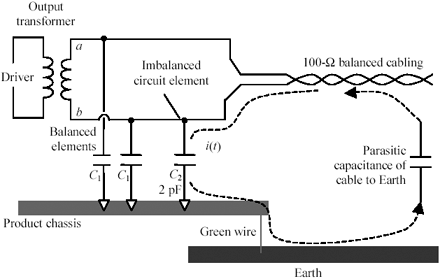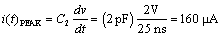# Differential to Common-Mode Conversion

Any imbalanced circuit element within an otherwise well-balanced transmission channel creates a region of partial coupling between the differential and common modes of transmission at that point. The coupling can translate part of a perfectly good differential signal into a common-mode signal, or vice versa.

Such differential-to-common mode conversion problems frequently arise in the design of LAN adapters. For example, assume the output winding of the transformer in Figure 6.9 has equal capacitances C 1 connected from point a to ground and from b to ground. If the capacitances are exactly equal (and the cable and transformer perfectly symmetrical), the differential signal present on the cable forces equal but opposite currents through these two capacitances. In the product chassis, the two currents perfectly cancel. The perfect cancellation implies that no current circulates between the twisted-pair cable and the surrounding chassis. In actual practice, however, one capacitance is always a little larger than the other.

Figure 6.9. An imbalanced circuit element within the transmitter causes current to circulate through the external cabling and the product chassis.Let capacitor C 2 in Figure 6.9 represent the small amount of physical imbalance (2 pF) between the parasitic capacitances associated with circuit nodes a and b . Let's calculate the current flowing through this capacitor, see where it flows, and then decide if it causes any problems.

Using Ethernet 10BASE-T for this example, the drive amplitude is approximately 2V p-p on each wire, at a switching time of 25 ns. The current forced through capacitor C 2 is

Equation 6.12This current flows through capacitor C 2 to the product chassis. It couples from the product chassis to the Earth (either through the green-wire ground or through the capacitance between the product chassis and the Earth). From the Earth it couples capacitively to the cabling, along which it travels as a common-mode signal riding on the twisted-pair cable back to the transformer, completing the loop.

A balanced load composed of equal-valued capacitors from a to ground and from b to ground would not generate any common-mode currents, because the currents through the two capacitors cancel, leaving nothing to exit the system in common-mode format. In this example the imbalance in capacitive loading generates the common-mode current.

A capacitive imbalance even as small as 2 pF causes a big problem in this example, because 160 m A of high-frequency common-mode current on an exposed cable easily violates U.S. and international emissions regulations.

POINT TO REMEMBER

• An imbalanced circuit can translate part of a perfectly good differential signal into a common-mode signal, or vice versa.High-Speed Signal Propagation[c] Advanced Black Magic
ISBN: 013084408X
EAN: N/A
Year: 2005
Pages: 163